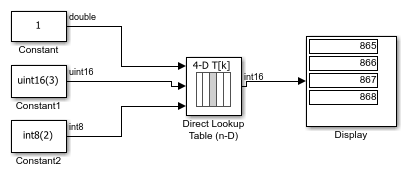Documentation

## Specifying Table Data in the Direct Lookup Table Block Dialog Box

This example shows how to specify table data on the dialog box of the Direct Lookup Table (n-D) block. In the following model, the table data is a is a 4-D array of linearly increasing values that you define with the following model preload function:

`a = reshape(1:2800, [4 5 20 7]);`

When you run the model, you get the following results:Because the Direct Lookup Table (n-D) block uses zero-based indexing, the output is:

`a(:,2,4,3)`

The output data type matches the Direct Lookup Table block's Table data type, which is set to `int16`.

Download now